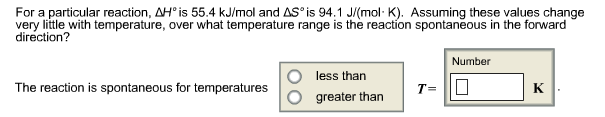# Problem: For a particular reaction, delta Hº is 55.4 kJ/mol and delta Sº is 94.1 J/(molK). Assuming these values change very little with temperature, over what temperature range is the reaction spontaneous in the forward direction?

###### FREE Expert Solution
83% (440 ratings)
###### FREE Expert Solution

Let's use Gibbs Free Energy Equation:
ΔG = ΔH - TΔS
Since the reaction is spontaneous, therefore ΔG < 0:

83% (440 ratings)###### Problem Details

For a particular reaction, delta Hº is 55.4 kJ/mol and delta Sº is 94.1 J/(molK). Assuming these values change very little with temperature, over what temperature range is the reaction spontaneous in the forward direction?What scientific concept do you need to know in order to solve this problem?

Our tutors have indicated that to solve this problem you will need to apply the Spontaneous Reaction concept. You can view video lessons to learn Spontaneous Reaction. Or if you need more Spontaneous Reaction practice, you can also practice Spontaneous Reaction practice problems.

What is the difficulty of this problem?

Our tutors rated the difficulty ofFor a particular reaction, delta Hº is 55.4 kJ/mol and delta...as medium difficulty.

How long does this problem take to solve?

Our expert Chemistry tutor, Sabrina took 3 minutes and 45 seconds to solve this problem. You can follow their steps in the video explanation above.

What professor is this problem relevant for?

Based on our data, we think this problem is relevant for Professor Daly's class at ARIZONA.